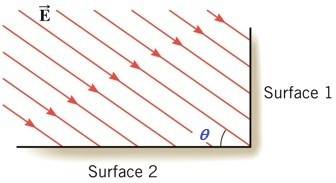# Electric flux?

## Homework Statement

The drawing shows an edge-on view of two planar surfaces that intersect and are mutually perpendicular. Surface (1) has an area of 2.20 m², while surface (2) has an area of 3.90 m². The electric field in the drawing is uniform and has a magnitude of 200 N/C. Find the magnitude of the electric flux through surface (2) if the angle θ made between the electric field with surface (2) is 35.0°.## Homework Equations

I used gausse law

## The Attempt at a Solution

(200)(2.20)cos(35)=360.4
(200)(3.90)cos(35)=638.9
Sinnce they are looking for magnitude of surface 2 I thought the answer was 638.9 but I got it wrong.[/B]

TSny
Homework Helper
Gold Member
Welcome to PF!

You used cos(35) when calculating the flux through surface 2. Think about whether or not you used the correct angle here.

what do u mean?

ok since it is a 90 degree angle then subtracting 35 should give me 55 right?

ok since it is a 90 degree angle then subtracting 35 should give me 55 right?
Yes, using Gauss' Law, EAcosθ, where θ refers to the angle between the electric field's direction and the line perpendicular to the area of application (which is the dot product of E and A, E⋅A)

thank u!!!!!!!!!!